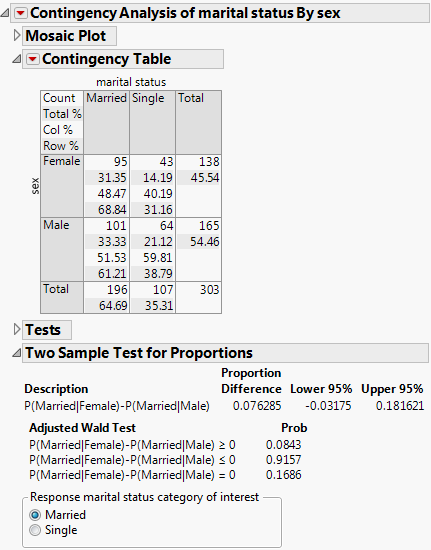Basic Analysis > Contingency Analysis > Additional Examples of the Contingency Platform > Example of a Two Sample Test for Proportions
Publication date: 11/29/2021

## Example of a Two Sample Test for Proportions

This example examines the probability of being married for males and females.

1. Select Help > Sample Data Library and open Car Poll.jmp.

2. Select Analyze > Fit Y by X.

3. Select marital status and click Y, Response.

4. Select sex and click X, Factor.

5. Click OK.

6. Click the red triangle next to Contingency Analysis of marital status By sex and select Two Sample Test for Proportions.

Figure 7.16 Example of the Two Sample Test for Proportions ReportIn this example, you are comparing the probability of being married between females and males. See the Row% in the Contingency Table to obtain the following:

P(Married|Female) = 0.6884

P(Married|Male) = 0.6121

The difference between these two numbers, 0.0763, is the Proportion Difference shown in the report. The two-sided confidence interval is [-0.03175, 0.181621]. The p-value by the adjusted Wald method corresponding to the confidence interval is 0.1686, which is close to the p-value (0.1665) by Pearson’s Chi-square test. Generally, Pearson’s Chi-square test is more popular than the modified Wald’s test for testing the difference of two proportions.